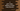# C program to print elements of an array and address of each element### C program to print array elements and address of each element :

In this tutorial, we will learn how to print the address and elements of an integer array. This example will show you how elements of an array are stored in memory . Let’s take a look at the program :

### C program :

``````#include <stdio.h>

int main()
{
//1
int myArray = {1, 2, 3, 4, 5, 6, 7, 8, 9};
int i;

//2
for (i = 0; i < 9; i++)
{
//3
printf("Address for %d is %d\n", myArray[i], &myArray[i]);
}
}``````

### Explanation :

The commented numbers in the above program denote the step number below :

1. Create one integer array myArray with some integer values. We will print these numbers and memory address where it is stored. Integer i is used in the loop below.
2. Run one for loop to read all numbers from the array. Print the number and its address. For printing the address we are using &myArray[i] for position i.

### Output :

``````Address for 1 is 1489689280